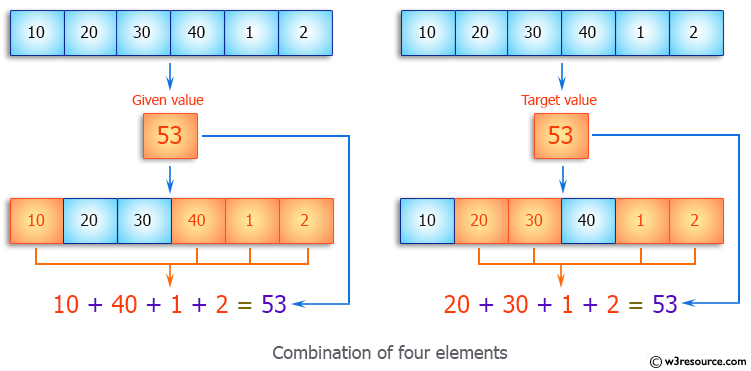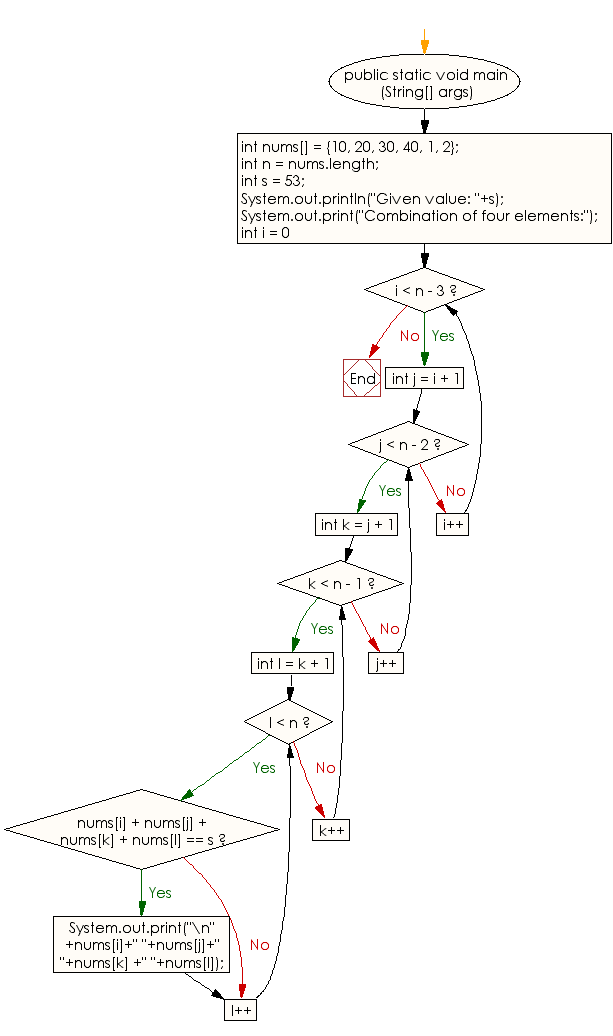﻿ Java: Combination of four elements of an array with same sum# Java Array Exercises: Find all combination of four elements of a given array whose sum is equal to a given value

## Java Array: Exercise-43 with Solution

Write a Java program to find all combination of four elements of a given array whose sum is equal to a given value.

Pictorial Presentation:Sample Solution:

Java Code:

``````import java.util.*;
import java.lang.*;
public class Main
{
public static void main (String[] args)
{
int nums[] = {10, 20, 30, 40, 1, 2};
int n = nums.length;
// given value
int s = 53;
System.out.println("Given value: "+s);
System.out.print("Combination of four elements:");
// Find other three elements after fixing first element
for (int i = 0; i < n - 3; i++)
{
// Find other two elements after fixing second element
for (int j = i + 1; j < n - 2; j++)
{
// Find the fourth element after fixing third element
for (int k = j + 1; k < n - 1; k++)
{
// find the fourth
for (int l = k + 1; l < n; l++)
{
if (nums[i] + nums[j] + nums[k] + nums[l] == s)
System.out.print("\n"+nums[i]+" "+nums[j]+" "+nums[k]
+" "+nums[l]);
}
}
}
}
}
}
```
```

Sample Output:

```
Given value: 53
Combination of four elements:
10 40 1 2
20 30 1 2
```

Flowchart:Visualize Java code execution (Python Tutor):

Java Code Editor:

Improve this sample solution and post your code through Disqus

What is the difficulty level of this exercise?

Test your Programming skills with w3resource's quiz.

﻿

## Java: Tips of the Day

System.currentTimeMillis vs System.nanoTime

In Java, there are two standard ways of conducting time-operations and it is not always clear which one should be chosen.

The method System.currentTimeMillis() returns the current number of milliseconds since the beginning of the Unix era in the format Long. Its accuracy ranges from 1 to 15 thousandths of a second, depending on the system.

`long startTime = System.currentTimeMillis();long estimatedTime = System.currentTimeMillis() - startTime;`

The method System.nanoTime has an accuracy of up to one-millionth of a second (nanoseconds) and returns the current value of the most accurate available system timer.

```long startTime = System.nanoTime();long estimatedTime = System.nanoTime() - startTime;
```

Thus, it is System.currentTimeMillisexcellent for displaying and synchronizing absolute time, and System.nanoTime for measuring relative intervals.

Ref: https://bit.ly/2W4V97w